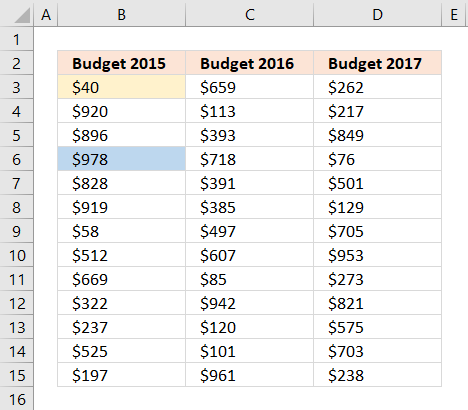Author: Oscar Cronquist Article last updated on November 17, 2021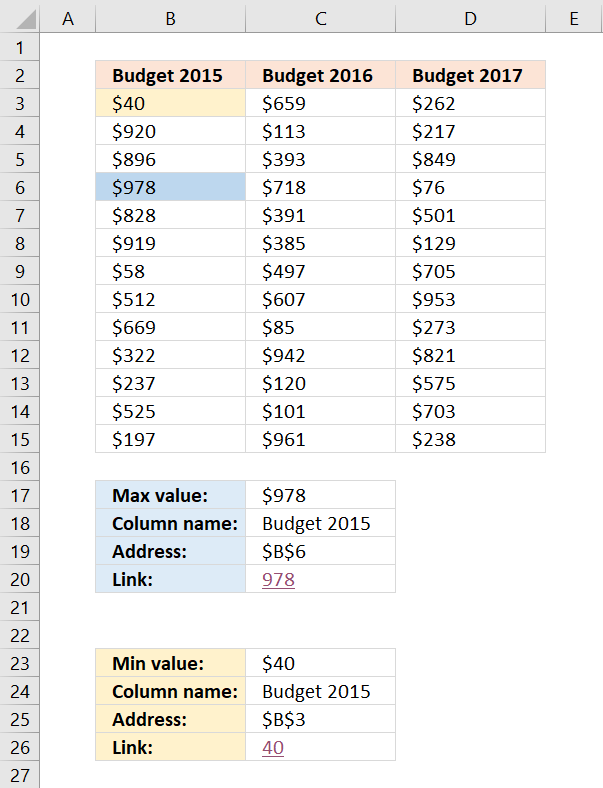This article demonstrates formulas that will return the largest and smallest numbers, the corresponding column headers, the cell addresses, and how to link to their location as well as highlighting those values.

Question: I have three columns and how do I identify the largest and smallest number? Where is the value?

I will now demonstrate four different formulas, all regular formulas that will tell you something about where the largest and smallest values are. I will also show you a technique that highlights the smallest and largest value.

## 1. Get the largest/smallest numberThe first formula returns the largest value from a cell range. The MAX function returns the largest number from a cell range.

Formula in cell C17:

=MAX(B3:D15)

This formula returns the smallest number:

=MIN(B3:D15)

### Explaining formula in cell C17

I am now going to explain the formula in cell C17: =MAX(B3:D15)

The MAX function returns the largest number in a cell range, you can also use multiple cell ranges, like this =MAX(B3:D15, F10:H22)

The MAX function allows you to have up to 255 arguments and it will ignore text and blank cells. However, if a cell contains an error value the function will also return an error value.

## 2. Get the column header name containing the largest/smallest numberThe following formula returns the header name of the column that contains the largest value. I will explain these formulas later on, in this article.

Formula in cell C18:

=INDEX(\$B\$2:\$D\$2, ,SUMPRODUCT((\$B\$3:\$D\$15=MAX(B3:D15))* MATCH(COLUMN(\$B\$3:\$D\$15), COLUMN(\$B\$3:\$D\$15))))

This formula returns the column header of the smallest number

=INDEX(\$B\$2:\$D\$2, ,SUMPRODUCT((\$B\$3:\$D\$15=MIN(B3:D15))* MATCH(COLUMN(\$B\$3:\$D\$15), COLUMN(\$B\$3:\$D\$15))))

### 2.1 Explaining formula in cell C18

I am now going to explain the formula in cell C18.

#### Step 1 - Get the largest number in a given cell range

The MAX function returns the largest number from a cell range or array.

MAX(B3:D15)

returns 978.

#### Step 2 - Where is the largest value?

The following logical expression returns an array that displays the location of the maximum value. This part of the formula compares the largest value to each value and returns TRUE if equal and FALSE if not.

The equal sign lets you compare values not considering upper and lower letters, use the EXACT function if you need to do a case sensitive comparison. The result is either boolean value TRUE or FALSE.

\$B\$3:\$D\$15=MAX(B3:D15)

becomes

\$B\$3:\$D\$15=978

becomes

{40, 659, 262;920, 113, 217;896, 393, 849;978, 718, 76;828, 391, 501;919, 385, 129;58, 497, 705;512, 607, 953;669, 85, 273;322, 942, 821;237, 120, 575;525, 101, 703;197, 961, 238}=978

and returns {FALSE, FALSE, FALSE;FALSE, FALSE, FALSE;FALSE, FALSE, FALSE;TRUE, FALSE, FALSE;FALSE, FALSE, FALSE;FALSE, FALSE, FALSE;FALSE, FALSE, FALSE;FALSE, FALSE, FALSE;FALSE, FALSE, FALSE;FALSE, FALSE, FALSE;FALSE, FALSE, FALSE;FALSE, FALSE, FALSE;FALSE, FALSE, FALSE}

The picture below shows the array in cell range F3:H15, the corresponding location in the array returns TRUE. Remaining values in the array show FALSE.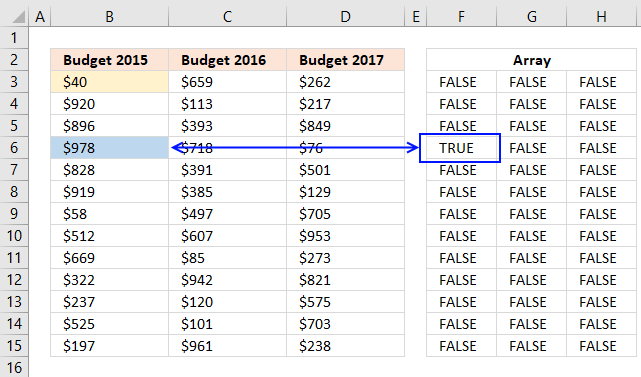#### Step 3 - Convert TRUE value to a column number

The next step is to multiply the array with the corresponding column numbers.

(\$B\$3:\$D\$15=MAX(B3:D15))* MATCH(COLUMN(\$B\$3:\$D\$15), COLUMN(\$B\$3:\$D\$15))

becomes

(\$B\$3:\$D\$15=MAX(B3:D15))* {1,2,3}

becomes

{FALSE, FALSE, FALSE;FALSE, FALSE, FALSE;FALSE, FALSE, FALSE;TRUE, FALSE, FALSE;FALSE, FALSE, FALSE;FALSE, FALSE, FALSE;FALSE, FALSE, FALSE;FALSE, FALSE, FALSE;FALSE, FALSE, FALSE;FALSE, FALSE, FALSE;FALSE, FALSE, FALSE;FALSE, FALSE, FALSE;FALSE, FALSE, FALSE}* {1,2,3}

and returns {0,0,0;0,0,0;0,0,0;1,0,0;0,0,0;0,0,0;0,0,0;0,0,0;0,0,0;0,0,0;0,0,0;0,0,0;0,0,0}

The picture below displays the array in cell range F3:H15, The TRUE value now contains number 1 which is the relative column number in cell range B3:D15 for that location. In other words, the maximum value is found in the first column.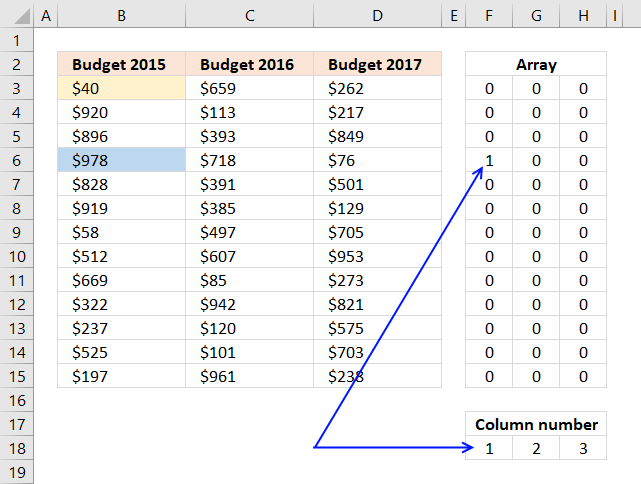#### Step 4 - Sum values in the array

The SUMPRODUCT function lets you do these mathematical operations without the need to enter the formula as an array formula.

SUMPRODUCT((\$B\$3:\$D\$15=MAX(B3:D15))* MATCH(COLUMN(\$B\$3:\$D\$15), COLUMN(\$B\$3:\$D\$15)))

becomes

SUMPRODUCT({0,0,0;0,0,0;0,0,0;1,0,0;0,0,0;0,0,0;0,0,0;0,0,0;0,0,0;0,0,0;0,0,0;0,0,0;0,0,0})

and returns 1.

#### Step 5 - Return header name

The INDEX function fetches a value based on coordinates. Since the values are in a single row we need only to enter the argument for the column number.

INDEX(cell_ref, row_number, column_number)

INDEX(\$B\$2:\$D\$2, ,SUMPRODUCT((\$B\$3:\$D\$15=MAX(B3:D15))* MATCH(COLUMN(\$B\$3:\$D\$15), COLUMN(\$B\$3:\$D\$15))))

becomes

INDEX(\$B\$2:\$D\$2, ,1)

becomes

INDEX({"Budget 2015","Budget 2016","Budget 2017"}, ,1)

and returns Budget 2015 in cell C18.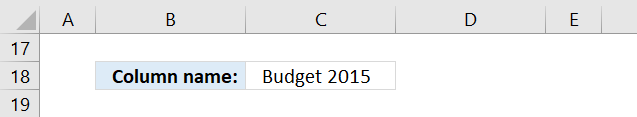### What happens if there are two or more cells containing the largest number?

The formula won't work, the sum will not correspond to the column numbers.

## 3. Get the cell address of the largest numberThis formula returns the address of the largest value, Excel has a grid with columns and rows. Example, address D16 is found in column D and row 16.

Formula in cell C19:

The following formula returns the address of the smallest number:

### 3.1 Explaining formula in cell C19

Step 1 to 4 calculate the row number used in the ADDRESS function and step 5 and 6 calculates the column number.

#### Step 1 - Get the largest number

The MAX function returns the largest number from a cell range or array.

MAX(B3:D15)

returns 978.

#### Step 2 - Identify where the largest number is

\$B\$3:\$D\$15=MAX(B3:D15)

becomes

\$B\$3:\$D\$15=978

becomes

{40, 659, 262; ... , 238}=978

and returns {FALSE, FALSE, FALSE; ... , FALSE}.

The arrays above are shorted, you can see the entire arrays in section 2.1.

#### Step 3 - Multiply with row numbers

The asterisk lets you multiply values, it works fine with boolean values as well as arrays.

(\$B\$3:\$D\$15=MAX(B3:D15))* ROW(\$B\$3:\$D\$15)

becomes

{FALSE, FALSE, FALSE;FALSE, FALSE, FALSE;FALSE, FALSE, FALSE;TRUE, FALSE, FALSE;FALSE, FALSE, FALSE;FALSE, FALSE, FALSE;FALSE, FALSE, FALSE;FALSE, FALSE, FALSE;FALSE, FALSE, FALSE;FALSE, FALSE, FALSE;FALSE, FALSE, FALSE;FALSE, FALSE, FALSE;FALSE, FALSE, FALSE}* {3; 4; 5; 6; 7; 8; 9; 10; 11; 12; 13; 14; 15}

and returns {0, 0, 0;0, 0, 0;0, 0, 0;6, 0, 0;0, 0, 0;0, 0, 0;0, 0, 0;0, 0, 0;0, 0, 0;0, 0, 0;0, 0, 0;0, 0, 0;0, 0, 0}.

The ROW function returns the row number of each row in B3:D15.

#### Step 4 - Sum numbers in array

The SUMPRODUCT function calculates the product of corresponding values and then returns the sum of each multiplication.

SUMPRODUCT(array1, [array2], ...)

SUMPRODUCT((\$B\$3:\$D\$15=MAX(B3:D15))* ROW(\$B\$3:\$D\$15))

becomes

SUMPRODUCT({0, 0, 0;0, 0, 0;0, 0, 0;6, 0, 0;0, 0, 0;0, 0, 0;0, 0, 0;0, 0, 0;0, 0, 0;0, 0, 0;0, 0, 0;0, 0, 0;0, 0, 0})

and returns 6.

#### Step 5 - Calculate column number

I showed how to calculate the largest number and how to compare the number to array \$B\$3:\$D\$15 in step 1 and 2.

(\$B\$3:\$D\$15=MAX(B3:D15))* COLUMN(\$B\$3:\$D\$15))

{FALSE, FALSE, FALSE;FALSE, FALSE, FALSE;FALSE, FALSE, FALSE;TRUE, FALSE, FALSE;FALSE, FALSE, FALSE;FALSE, FALSE, FALSE;FALSE, FALSE, FALSE;FALSE, FALSE, FALSE;FALSE, FALSE, FALSE;FALSE, FALSE, FALSE;FALSE, FALSE, FALSE;FALSE, FALSE, FALSE;FALSE, FALSE, FALSE}* {1, 2, 3}

and returns

{0, 0, 0;0, 0, 0;0, 0, 0;1, 0, 0;0, 0, 0;0, 0, 0;0, 0, 0;0, 0, 0;0, 0, 0;0, 0, 0;0, 0, 0;0, 0, 0;0, 0, 0}.

The COLUMN function returns a relative number for each column in cell range B3:B15.

#### Step 6 - Sum numbers in array

The SUMPRODUCT function calculates the product of corresponding values and then returns the sum of each multiplication.

SUMPRODUCT(array1, [array2], ...)

SUMPRODUCT((\$B\$3:\$D\$15=MAX(B3:D15))* COLUMN(\$B\$3:\$D\$15))

becomes

SUMPRODUCT({0, 0, 0;0, 0, 0;0, 0, 0;1, 0, 0;0, 0, 0;0, 0, 0;0, 0, 0;0, 0, 0;0, 0, 0;0, 0, 0;0, 0, 0;0, 0, 0;0, 0, 0})

and returns 1.

#### Step 7 - Get address based on row and column numbers

The ADDRESS function returns a cell reference as a text value, to be able to do that it needs to know the row and column number.

becomes

and returns \$B\$6 in cell C19.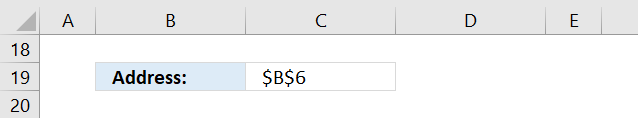## 4. Create a link to the largest numberThe next formula creates a hyperlink that allows you to quickly move to and select the largest value. You simply press with left mouse button on the cell and Excel instantly navigates to that cell, even if it is on another worksheet.

Formula in cell C20:

This formula returns a hyperlink pointing to the smallest number:

### 4.1 Explaining formula in cell C20

#### Step 1 - Calculate cell address

This part of the formula is already explained in section 3.1 above, check it out if you want a detailed explanation.

returns B6.

#### Step 2 - Create a hyperlink

This section explains the formula in cell C20. The HYPERLINK function allows you to create a link to a cell location. (It can do more than that but this is all you need to know for now.)

The link location is a cell reference to a specific cell in your workbook. You need to specify the workbook name, worksheet name, and cell address. Read the previous explanation to learn how to calculate the cell reference of the maximum value.

becomes

HYPERLINK("[Min and max out of multiple columns.xlsx]Sheet1!"&B6, MAX(B3:D15))

The second argument is the value that you want the cell to show, in this case, the maximum value.

HYPERLINK("[Min and max out of multiple columns.xlsx]Sheet1!"&B6, MAX(B3:D15))

becomes

HYPERLINK("[Min and max out of multiple columns.xlsx]Sheet1!"&B6, 978)

and returns 978 (hyperlink) in cell C20.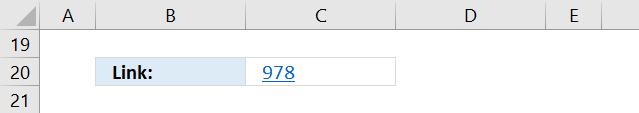## 5. How to highlight the largest and smallest number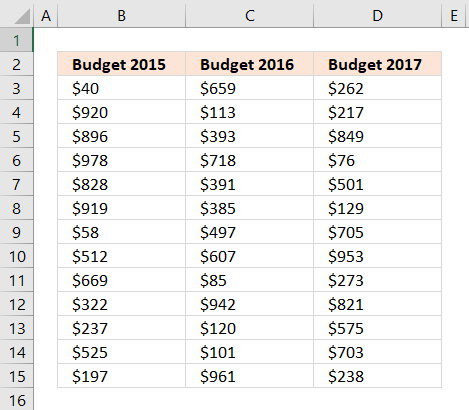Conditional formatting allows you to format specific values using a formula or built-in criteria, in this case, I will highlight the cells containing the maximum and minimum value in a cell range.

1. Select cell range B3:D15.
2. Go to tab "Home" if you are not already there.
3. Press with mouse on the "Conditional formatting" button.
4. Press with left mouse button on "New Rule..".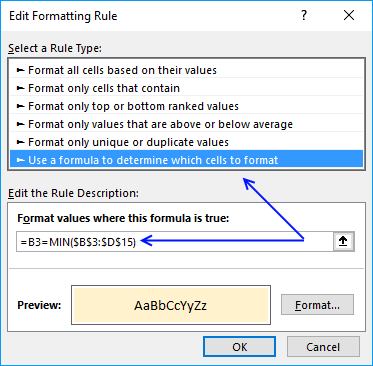5. Press with mouse on "Use a formula to determine which cells to format".
6. Type this formula in field "Format values where this formula is true:": =B3=MAX(\$B\$3:\$D\$15)
7. Press with left mouse button on "Format..." button.
8. Press with mouse on tab "Fill".
9. Pick a color.
10. Press with left mouse button on "OK" button.
11. Press with left mouse button on "OK" button.

Repeat the above steps to highlight the minimum value using this formula: =B3=MIN(\$B\$3:\$D\$15)
Remember to pick a different color.